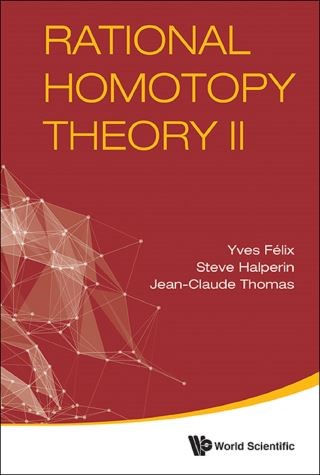## Rational Homotopy Theory Ii• Description
• Author
• Info
• Reviews

### Description

This research monograph is a detailed account with complete proofs of rational homotopy theory for general non-simply connected spaces, based on the minimal models introduced by Sullivan in his original seminal article. Much of the content consists of new results, including generalizations of known results in the simply connected case. The monograph also includes an expanded version of recently published results about the growth and structure of the rational homotopy groups of finite dimensional CW complexes, and concludes with a number of open questions. This monograph is a sequel to the book Rational Homotopy Theory [RHT], published by Springer in 2001, but is self-contained except only that some results from [RHT] are simply quoted without proof.Contents:

• Basic Definitions and Constructions
• Homotopy Lie Algebras and Sullivan Lie Algebras
• Fibrations and Λ-Extensions
• Holonomy
• The Model of the Fibre is the Fibre of the Model
• Loop Spaces and Loop Space Actions
• Sullivan Spaces
• Examples
• Lusternik-Schnirelmann Category
• Depth of a Sullivan Algebra and of a Sullivan Lie Algebra
• Depth of a Connected Graded Lie Algebra of Finite Type
• Trichotomy
• Exponential Growth
• Structure of a Graded Lie Algebra of Finite Depth
• Weight Decompositions of a Sullivan Lie Algebra
• Problems
• Key Features:
• Contains the basis for using rational homotopy theory for non-simply connected spaces
• Contains new important information on the rational homotopy Lie algebra of spaces
• Is at the frontier of the research in rational homotopy